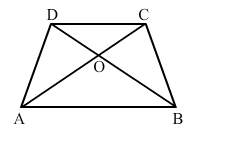# In the adjoining figure, ABCD is a trapezium in which CD ∥ AB and its diagonals intersect at O.Question:

In the adjoining figure, ABCD is a trapezium in which CD ∥ AB and its diagonals intersect at O. If AO = (2x + 1) cm, OC = (5x – 7) cm, DO = (7x − 5) cm and OB = (7x + 1) cm, find the value of x.Solution:

In trapezium $\mathrm{ABCD}, A B \| C D$ and the diagonals $\mathrm{AC}$ and $\mathrm{BD}$ intersect at $\mathrm{O}$.

Therefore,

$\frac{A O}{O C}=\frac{B O}{O D}$

$\Rightarrow \frac{2 x+1}{5 x-7}=\frac{7 x+1}{7 x-5}$

$\Rightarrow(5 x-7)(7 x+1)=(7 x-5)(2 x+1)$

$\Rightarrow 35 x^{2}+5 x-49 x-7=14 x^{2}-10 x+7 x-5$

$\Rightarrow 21 x^{2}-41 x-2=0$

$\Rightarrow 21 x^{2}-42 x+x-2=0$

$\Rightarrow 21 x(x-2)+1(x-2)=0$

$\Rightarrow(x-2)(21 x+1)=0$

$\Rightarrow x=2,-\frac{1}{21}$

$\because x \neq-\frac{1}{21}$

$\therefore x=2$